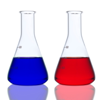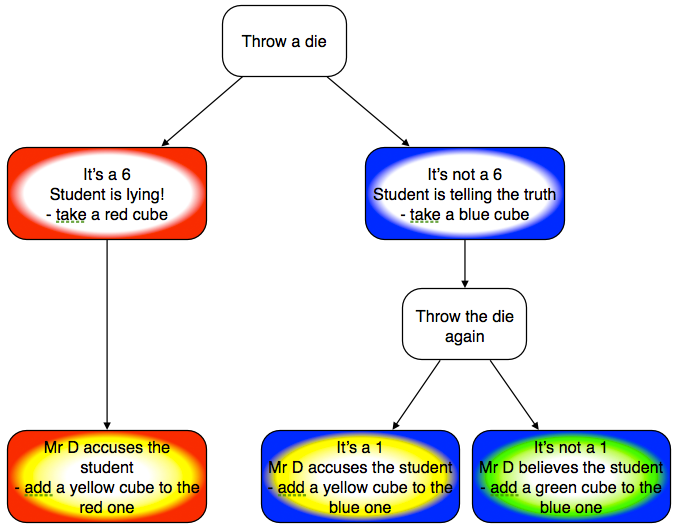You may also likeWhich Team Will Win?

A practical experiment which will introduce students to tree diagrams, and help them to understand that outcomes may not be equally likely.Who Is Cheating?

A practical experiment provides data. Moving onto expected results provides a context to establish the multiplication rule in probability, and an intuitive approach to conditional probability.

The Dog Ate My Homework!

Age 11 to 16Challenge Level
This problem is part of the Great Expectations: Probability through Problems collection. It is designed for classroom use; see the Teachers' Resources for a suggested classroom approach.A certain teacher, Mr L I Detector, claims he can tell when students are lying about their homework.

This is true.

Unfortunately, he also accuses some students who are telling the truth.

So what are the chances that someone who is accused is actually telling the truth?

You can investigate this question through a practical experiment.
You will need a 6-sided die, and some red, blue, green and yellow multi-link cubes.
The red and blue cubes represent whether a student is telling the truth or lying.
The yellow and green cubes represent whether Mr L I Detector accuses a student or not.

First investigate what happens for one student:What do each of these mean?
• red and yellow
• blue and yellow
• blue and green
Why can't you have red and green?

Now repeat the experiment 36 times in total.  You should end up with 36 pairs of cubes.

Are you surprised by your results?

How do they compare with what you would expect?  (You may find this worksheet helpful here).

What proportion of truthful students are accused?  So what is the probability of a truthful student being accused?

What proportion of accused students are telling the truth?

Is the probability that a truthful student is accused the same as the probability that an accused student is telling the truth? Are you surprised by this?

You can explore the scenario in this problem using the interactive environment.